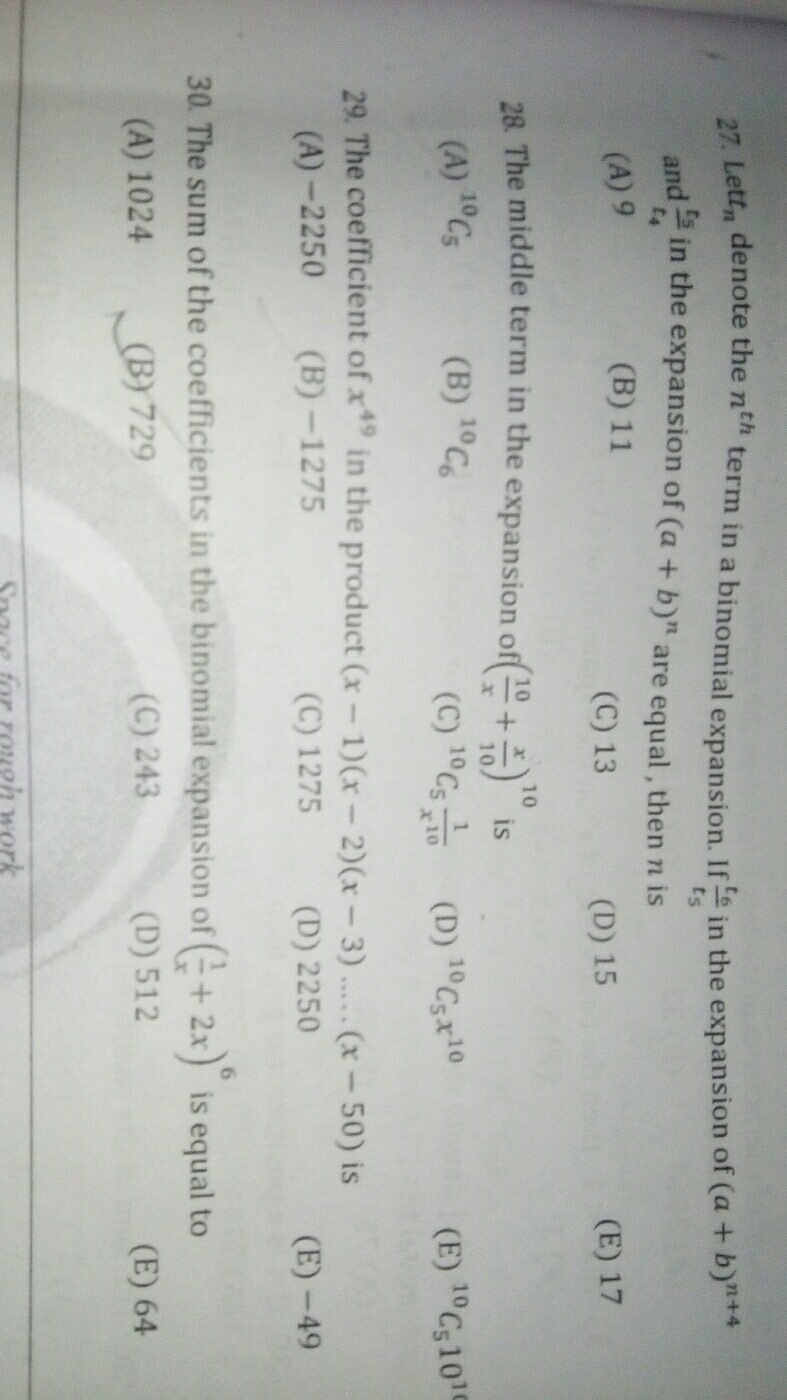S
Sudeeptvd
10 Apr 18

How to solve question 29?## Replies to this post

S
Sudeeptvd

0
A
Anju

The expansion will be x^50 +a1x^49+a2x^48+......+a49x+a50 =0
The roots of the equation are 1,2,3,4....50
a1 is the minus of the sum of roots of the equation.
a1=-(1+2+3+...50)
=-1275

0
A
Anju

From the question you can find that the roots of the equation will be 1,2,3,...50
Coefficient of x^49 is a1.
You can use a trick here, consider(x-a)(x-b)= x^2- (a+b)x +b^2
Similarly
x^50-(1+2+3+4+.....49)x^49+....... will be the expansion. The coefficient of x^49 will be sum of 1 to 49

0